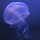## General Question# What is the difference between gravitational mass and inertial mass?

Asked by VanBantam (161) June 11th, 2008

Ok guys here’s a real screw ball. I’ve thought about this a bit but would like to see what others think. So I’d like to hear from all of you: non-physicists, armchair physicists, amature physicists, physicists without degrees, and physicists with PhDs.
The problem: the mass term used in Newton’s laws of gravitiation is not necissarily the same as the mass term used in his second law of motion. If they are different why? If the are the same why?

Observing members: 0Composing members: 0In classical physics, the two were theoretically different because Newton’s formulas were really only approximations for large bodies. Inertial and gravitational mass are experientially the same, but Newton doesn’t require them to be the same. This was reconciled in special relativity, however. In fact, the equivalency of inertial and gravitational mass is one of the main underpinnings of general relativity.

Einstein argued that the differences between the two are based on frames of reference and a misunderstanding of gravity in classical physics and that, in fact, the two have to be the same.

If you want a more detail understanding, the Wikipedia article on the equivalence principle is a pretty good overview.

soundedfury (2536)“Great Answer” (5) Flag as…or